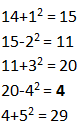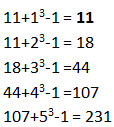# Number Series Quiz for ISRO Assistant Exam#### Q.1 14, 15, 11, 20, ?, 29

A. 6
B. 25
C. 4
D. 20
E. None of these
Ans. C.
Solution:A. 4
B. 5
C. 6
D. 8
E. 10
Ans. A.
Solution:
6×.5+1 = 4
4×1+2 = 6
6×2+4 = 16
16×4+8 = 72
72×8+16 = 592

A. 633
B. 599
C. 613
D. 512
E. 603
Ans. E.
Solution:
14+19 = 33
33+38 = 7
71+76= 147
147+152 = 299
299+304 = 603

A. 165
B. 185
C. 175
D. 195
E. 205
Ans. C.
Solution:
9×1-1 = 8
8×2-1 = 15
15×3-1 = 44
44×4-1 = 175
175×5-1 = 874

A. 50
B. 35
C. 42
D. 39
E. 48
Ans. D.
Solution:
3+4 = 7
7+4+2 = 13
13+6+4 = 23
23+10+6 = 39
39+16+8 = 63

#### Q.6 11, ?, 18, 44, 107, 231

A. 15
B. 11
C. 12
D. 14
E. 10
Ans. B.
Solution:A. 16
B. 8
C. 12
D. 20
E. 40
Ans. A.
Solution:
1×16 = 16
16×8 = 128
128×4 = 512
512×2 = 1024
1024×1 = 1024

A. 865
B. 795
C. 891
D. 720
E. 841
Ans. C.
Solution:
3×1+2 = 5
5×2+3 = 13
13×3+4 = 43
43×4+5 = 177
177×5+6 = 891

A. 14
B. 12
C. 16
D. 18
E. None of these
Ans. A.
Solution:
126÷2-1 = 62
62÷2-1 = 30
30÷2-1 = 14
14÷2-1 = 6
6÷2-1 = 2

#### Q.10 34, 40, 42, 32, ?, 24

A. 42
B. 55
C. 45
D. 50
E. None of these
Ans. D.
Solution:
There are 2 series in the given question:
34, 42, ?
34+8 = 42
42+8 = 50
40, 32, 24
40-8 = 32
32-8 = 24#### What's trending in BankExamsToday

Smart Prep Kit for Banking Exams by Ramandeep Singh - Download here1.didn't get the logic

Q.5 3, 7, 13, 23, ?, 63

A. 50
B. 35
C. 42
D. 39
E. 48
Ans. D.
Solution:
3+4 = 7
7+4+2 = 13
13+6+4 = 23
23+10+6 = 39
39+16+8 = 63

2.thanks sir ji https://careerwing.in

Thanks for commenting. Follow us on Telegram. Search BankExamsToday